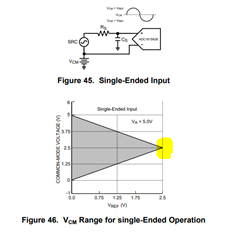If you have a related question, please click the "Ask a related question" button in the top right corner. The newly created question will be automatically linked to this question.

Hi team,

If the ADC161S626 is been driven by a singe-ended source as below shown, and when Vref=2.5V,Vcm =2.5V,  would the output of ADC161S626 be distorted?

Thank you!Regards,
Ivy
• Hello Ivy,

In single ended configuration, the ADC161S626 will have slightly degraded performance verses driving the input fully differential, but the part will still perform very well.

Regards,
Keith Nicholas

• Hi Keith,

Can I understand if the Vref=2.5V, the Vcm can't exceed 2.5V or below 2.5V, if over the Vcm range(shaded in the figure), what will the output?

Regards,

Ivy

• Hello Ivy,

The +IN analog input is limited from 0V to VA, which is typically equal to 5V.  Since the full scale input range [Vdiff=(+IN)-(-IN)] is from -Vref to +Vref, and Vref=2.5V, then the only level for -IN pin that supports the full range is -IN=Vcm=2.5V.

You can change the voltage on the -IN pin and this will limit the full scale input range that can be measured.

Example 1: Vref=2.5V, Vcm=4V

+IN=5V, Vdiff:max=(5-4)=+1V

+IN=1.5V, Vdiff:min=(1.5-4)=-2.5V

In this case, you will lose some of your full scale input range because the maximum differential input voltage that can be measured is +1V.

Example 2: Vref=2.5V, Vcm=1.5V

+IN=4V, Vdif:maxf=(4-1.5)=+2.5V

+IN=0V, Vdiff:min=(0-1.5)=-1.5V

In this case, you also lose some of your full scale input range because the minimum differential input voltage that can  be measured is -1.5V.

I hope this helps!

Regards,
Keith

• Hi Keith，

The common mode voltage Vcm shown in Figure 46 is not the same as the actual calculated Vcm', which I have some doubts about. Could you please help give some comments? Thank you.

Example 1: Vref=2.5V, Vcm=4V

-IN=Vcm=4V

+IN max=VA=5V

Actual Vcm'=[(-IN)+(+IN)]/2=4.5V>Vcm(4V)

Regards,

Ivy

• Hello Ivy,

Yes, this is confusing.  The datasheet is referring to the voltage applied to the (-IN) pin as the common mode voltage, which is not technically correct.

As you pointed out, the actual definition of Vcm should be [(-IN)+(+IN)]/2, but in this older datasheet, Vcm is simply equal to the voltage applied to (-IN) pin.

I hope this helps clear thinks up.

Regards,
Keith